Home > INT3 > Chapter 11 > Lesson 11.1.3 > Problem11-34

11-34.
1. Multiply or divide the expressions below. Leave your answers as simplified as possible. Homework Help ✎

2.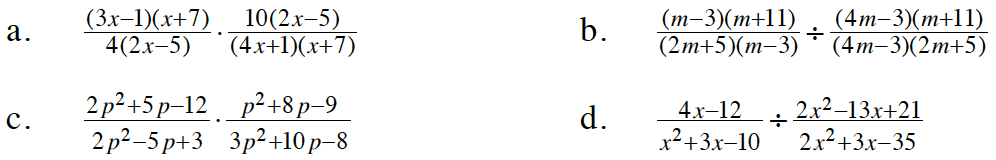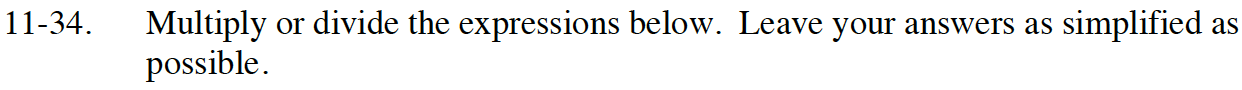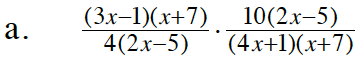Look for Giant Ones. Does anything factor out?

Remember, 10 and 4 have a common factor, too.

$\frac{5(3x-1)}{2(4x+1)}$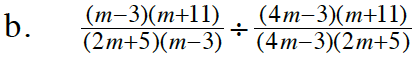Take the reciprocal of the divisor (the fraction on the right), and multiply the resulting fractions. Does anything factor out before you begin multiplying?

1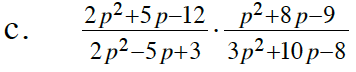Refer to part (a). Factor each of the trinomials first. (Use a generic rectangle.)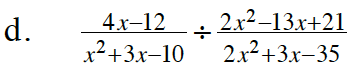4x − 12 can be factored, too.

$\frac{4}{x-2}$